Next: Histogram Bin Size Up: Implementation Previous: Implementation

## Parameterisation

The first implementation choice is the way in which the transformation is represented. A simple, but poor, choice is to use one parameter for each entry in the first three rows of the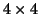affine matrix. However, this highly couples the parameters which makes it very difficult for Powell's method to work efficiently. In addition, the matrix elements themselves are not particularly meaningful. Therefore, the best parameterisation is one that decouples the parameters in a sensible way.

One standard decomposition of an affine matrix, which decouples the parameters, is to split the transformation into 3 rotation, 3 translation, 3 scale and 3 skew parameters. That is: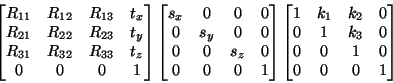(36)

where the product is theaffine matrix, Rijrepresents the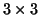rotation matrix, ti represents a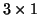translation vector, si are the scale parameters, and ki are the skew parameters. It is also necessary to further decompose the rotation matrix into three independent parameters such as Euler angles which represent a rotation by three independent rotations, each about one of the coordinate axes: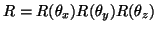.

This fully accounts for the 12 Degrees Of Freedom and allows simple restrictions to be placed on the parameters, such as sx = sy = sz. Consequently, the reduced 9, 7 and 4 DOF optimisations required by the method outlined in the previous section are straightforward to implement.

These parameters, however, are still not fully specified as the decomposition can be performed about any given origin. This origin functions as the centre of rotations, scales and skews, and affects the coupling between these parameters and the translation parameters. By choosing the origin to be the Centre of Mass for the volume, the coupling is minimised in the sense that applying a rotation to some hypothesized alignment results in a transformation where the translation is still approximately correct, as opposed to choosing the origin at the corner of the image where applying a rotation will significantly affect the overlap.

The Centre of Mass (COM) is defined by taking the intensity as a mass density (or the intensity less the minimum intensity in order to assure that it is positive) so that: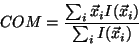(37)

where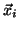are the 3D spatial locations of the lattice sites.

For example, consider an ellipsoid in the reference and floating image that is almost aligned correctly, except for a small rotation, see figure 5. Applying the correct adjustment in rotation but using an origin at the corner of the volume will cause the orientation of the two ellipsoids to be correct but will result in a translational error. Therefore, to find the correct transformation using this parameterisation requires first one rotation parameter to be adjusted and then all three translation parameters. However, because the cost function will be greatly affected by the amount of overlap of the images, the minima of cost as the rotation is varied is largely going to reflect the change in position (translation) rather than the change in orientation (rotation). On the other hand, by having the origin at the COM, the rotation change does not affect the translation greatly, and a search along each parameter independently is much more likely to find the correct solution.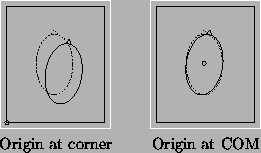Even with this choice of origin there is coupling between the translation and remaining parameters. Furthermore, as Powell's method is capable of estimating linear parameter couplings it might seem that decoupling the parameters initially is unimportant. However, these couplings are non-linear and therefore beyond the scope of Powell's method to estimate.

In addition, by taking the origin at the COM, the identity transformation will align the COM of the two volumes, which makes this a sensible choice for any initial transformation.Next: Histogram Bin Size Up: Implementation Previous: Implementation
Mark Jenkinson
2000-05-10